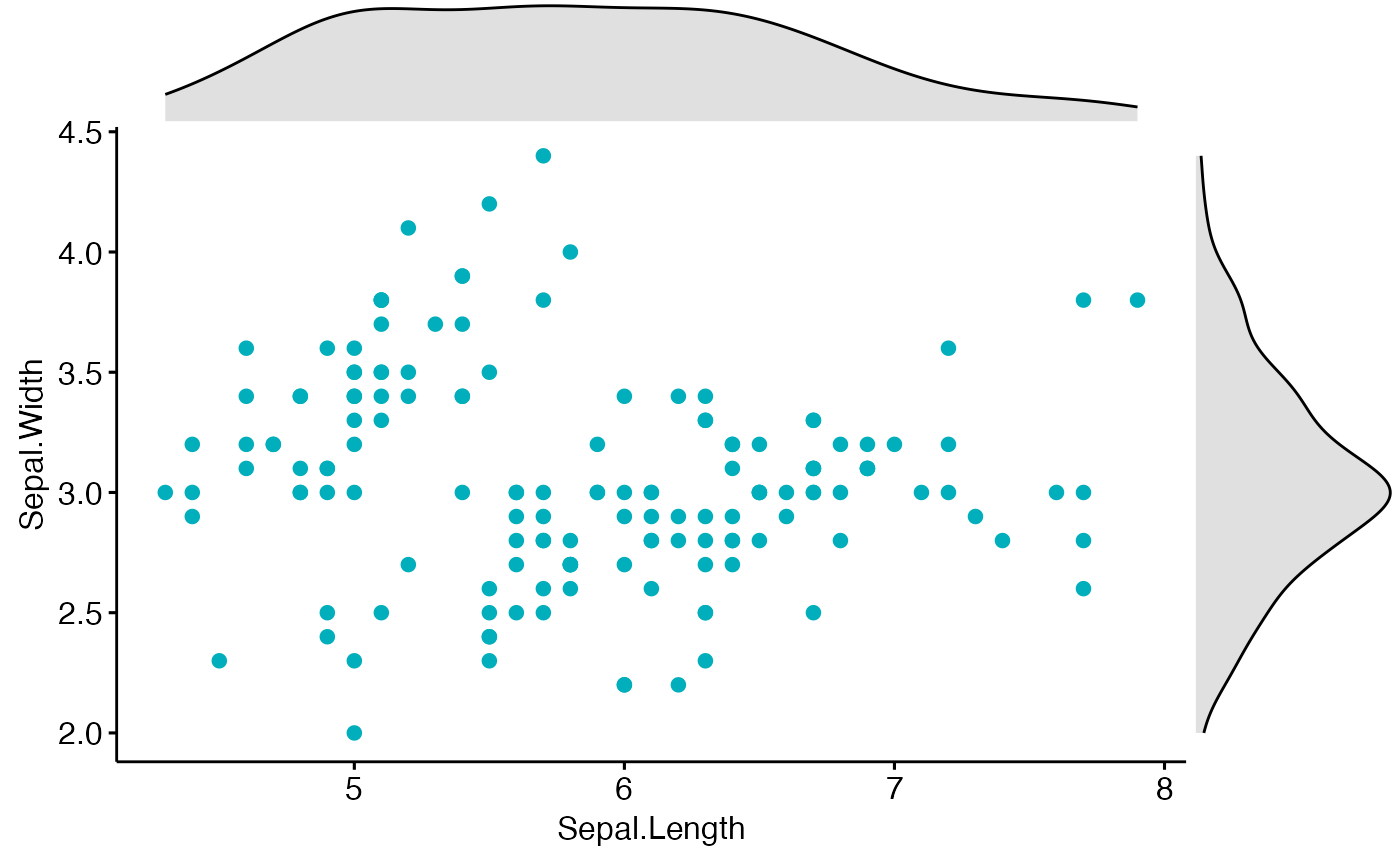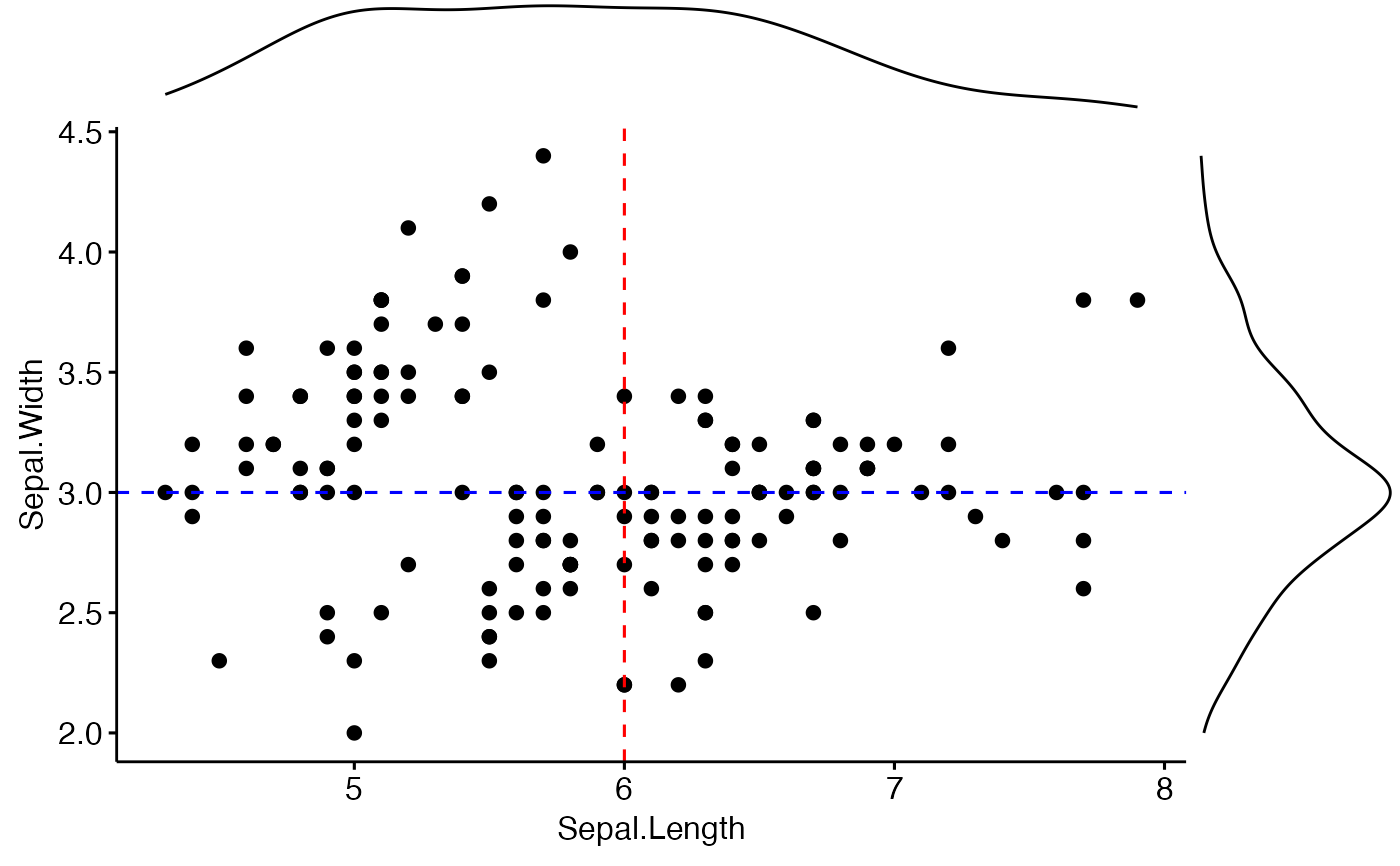Create a scatter plot with marginal histograms, density plots or box plots.

ggscatterhist(
data,
x,
y,
group = NULL,
color = "black",
fill = NA,
palette = NULL,
shape = 19,
size = 2,
linetype = "solid",
bins = 30,
margin.plot = c("density", "histogram", "boxplot"),
margin.params = list(),
margin.ggtheme = theme_void(),
margin.space = FALSE,
main.plot.size = 2,
margin.plot.size = 1,
title = NULL,
xlab = NULL,
ylab = NULL,
legend = "top",
ggtheme = theme_pubr(),
print = TRUE,
...
)

# S3 method for ggscatterhist
print(
x,
margin.space = FALSE,
main.plot.size = 2,
margin.plot.size = 1,
title = NULL,
legend = "top",
...
)

## Arguments

data a data frame an object of class ggscatterhist. x and y variables for drawing. a grouping variable. Change points color and shape by groups if the options color and shape are missing. Should be also specified when you want to create a marginal box plot that is grouped. point colors. point colors. the color palette to be used for coloring or filling by groups. Allowed values include "grey" for grey color palettes; brewer palettes e.g. "RdBu", "Blues", ...; or custom color palette e.g. c("blue", "red"); and scientific journal palettes from ggsci R package, e.g.: "npg", "aaas", "lancet", "jco", "ucscgb", "uchicago", "simpsons" and "rickandmorty". point shape. See show_point_shapes. Numeric value (e.g.: size = 1). change the size of points and outlines. line type ("solid", "dashed", ...) Number of histogram bins. Defaults to 30. Pick a better value that fit to your data. the type of the marginal plot. Default is "hist". parameters to be applied to the marginal plots. the theme of the marginal plot. Default is theme_void(). logical value. If TRUE, adds space between the main plot and the marginal plot. the width of the main plot. Default is 2. the width of the marginal plot. Default is 1. plot main title. character vector specifying x axis labels. Use xlab = FALSE to hide xlab. character vector specifying y axis labels. Use ylab = FALSE to hide ylab. specify the legend position. Allowed values include: "top", "bottom", "left", "right". the theme to be used for the scatter plot. Default is theme_pubr(). logical value. If TRUE (default), print the plot. other arguments passed to the function ggscatter().

## Value

an object of class ggscatterhist, which is list of ggplots, including the following elements:

• xplot: marginal x-axis plot;

• yplot: marginal y-axis plot.

.

User can modify each of plot before printing.

## Examples

# Basic scatter plot with marginal density plot
ggscatterhist(iris, x = "Sepal.Length", y = "Sepal.Width",
color = "#00AFBB",
margin.params = list(fill = "lightgray"))# Grouped data
ggscatterhist(
iris, x = "Sepal.Length", y = "Sepal.Width",
color = "Species", size = 3, alpha = 0.6,
palette = c("#00AFBB", "#E7B800", "#FC4E07"),
margin.params = list(fill = "Species", color = "black", size = 0.2)
)# Use boxplot as marginal
ggscatterhist(
iris, x = "Sepal.Length", y = "Sepal.Width",
color = "Species", size = 3, alpha = 0.6,
palette = c("#00AFBB", "#E7B800", "#FC4E07"),
margin.plot = "boxplot",
ggtheme = theme_bw()
)# Add vertical and horizontal line to a ggscatterhist
plots <- ggscatterhist(iris, x = "Sepal.Length", y = "Sepal.Width", print = FALSE)
plots$sp <- plots$sp +
geom_hline(yintercept = 3, linetype = "dashed", color = "blue") +
geom_vline(xintercept = 6, linetype = "dashed", color = "red")
plots